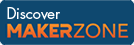Cody

# Problem 37. Pascal's Triangle

Created by Cody Team in Community

Given an integer n >= 0, generate the length n+1 row vector representing the n-th row of Pascal's Triangle.

Examples:

``` pascalTri(0)
ans =
1```
``` pascalTri(1)
ans =
1     1```
``` pascalTri(2)
ans =
1     2     1```

### Solution Stats

50.45% Correct | 49.55% Incorrect
Last solution submitted on Mar 18, 2019

#### TagsMATLAB and Simulink resources for Arduino, LEGO, and Raspberry Pi